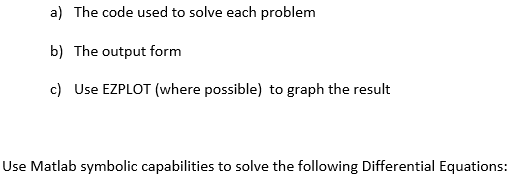# How graph second order differential equation with Matlab?

33 ビュー (過去 30 日間)
jake thompson 2018 年 1 月 18 日
Commented: Rena Berman 2019 年 12 月 12 日
Looking to get some help on how to use matlab to solve and plot the following equation, all help is appreciated! Thank You! I haven't used matlab in 2 years very rusty, image is reference to original problem statement.x^2*y"-7xy'+16y=0 & y*y"=2y'^2

#### 1 件のコメント

Rena Berman 2019 年 12 月 12 日

サインイン to comment.

### 件の回答 (1)

Star Strider 2018 年 1 月 18 日
Do them the same as you did before. Define ‘Dy’ and ‘D2y’, then substitute them for y' and y" respectively. Use both of them in your dsolve call, separated by commas, and include the requisite initial conditions, as I did. See the dsolve documentation for details,

#### 2 件のコメント

jake thompson 2018 年 1 月 18 日
I did it like the previous problem, this is my code. When I run it I get an error.
clear; syms y(x)
Dy = diff(y);
D2y = diff(y,2);
ode = x^2*D2y - 7*x*Dy + 16*y == 0;
ySol = dsolve(ode, y(0) == 0, Dy(0) == 1)
figure
ezplot(ySol)
Warning: Explicit solution could not be found. > In dsolve (line 201)
ySol =
[ empty sym ]
Star Strider 2018 年 1 月 18 日
I didn’t take a close look at it. You have two equations, so you need to code both of them.
It appears to be a system of nonlinear differential equations. Most nonlinear differential equations do not have analytic solutions. It would be necessary to solve them numerically.
The best way to do that is to use the odeToVectorFiled function first, then matlabFunction on that result, to get an anonymous function to integrate with one of the numerical ODE solvers, such as ode45.
Prototype code for that:
[V,S] = odeToVectorField(ode1,ode2)
M = matlabFunction(V,'vars',{'t','Y'})
You would then use ‘M’ with ode45.

サインイン to comment.

サインイン してこの質問に回答します。